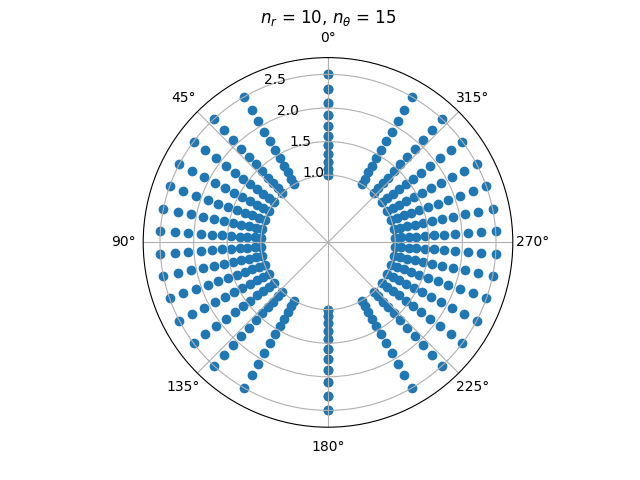pfsspy magnetic field grid

A plot of the grid corners, from which the magnetic field values are taken when tracing magnetic field lines.

Notice how the spacing becomes larger at the poles, and closer to the source surface. This is because the grid is equally spaced in $$\cos \theta$$ and $$\log r$$.

import matplotlib.pyplot as plt
import numpy as np

from pfsspy.grid import Grid

Define the grid spacings

ns = 15
nphi = 360
nr = 10

Create the grid

grid = Grid(ns, nphi, nr, rss)

Get the grid edges, and transform to r and theta coordinates

r_edges = np.exp(grid.rg)
theta_edges = np.arccos(grid.sg)

The corners of the grid are where lines of constant (r, theta) intersect, so meshgrid these together to get all the grid corners.

r_grid_points, theta_grid_points = np.meshgrid(r_edges, theta_edges)

Plot the resulting grid corners

fig = plt.figure()

ax.scatter(theta_grid_points, r_grid_points)
ax.scatter(theta_grid_points + np.pi, r_grid_points, color='C0')

ax.set_title('$n_{r}$ = ' f'{nr}, ' r'$n_{\theta}$ = ' f'{ns}')